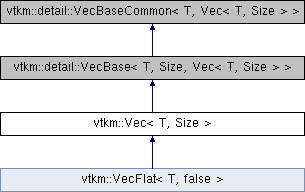VTK-m  2.0
vtkm::Vec< T, Size > Class Template Reference

A short fixed-length array. More...

`#include <Types.h>`

Inheritance diagram for vtkm::Vec< T, Size >:## Public Types

using ComponentType = T

## Public Member Functions

Vec ()=default

VTKM_EXEC_CONT void CopyInto (Vec< T, Size > &dest) const

## Static Public Attributes

static constexpr vtkm::IdComponent NUM_COMPONENTS = Size

## Private Types

using Superclass = detail::VecBase< T, Size, Vec< T, Size > >

## Detailed Description

### template<typename T, vtkm::IdComponent Size> class vtkm::Vec< T, Size >

A short fixed-length array.

The `Vec` templated class holds a short array of values of a size and type specified by the template arguments.

The `Vec` class is most often used to represent vectors in the mathematical sense as a quantity with a magnitude and direction. Vectors are, of course, used extensively in computational geometry as well as physical simulations. The `Vec` class can be (and is) repurposed for more general usage of holding a fixed-length sequence of objects.

There is no real limit to the size of the sequence (other than the largest number representable by vtkm::IdComponent), but the `Vec` class is really designed for small sequences (seldom more than 10).

## ◆ ComponentType

template<typename T , vtkm::IdComponent Size>
 using vtkm::Vec< T, Size >::ComponentType = T

## ◆ Superclass

template<typename T , vtkm::IdComponent Size>
 using vtkm::Vec< T, Size >::Superclass = detail::VecBase >
private

## ◆ Vec()

template<typename T , vtkm::IdComponent Size>
 vtkm::Vec< T, Size >::Vec ( )
default

## ◆ CopyInto()

template<typename T , vtkm::IdComponent Size>
 VTKM_EXEC_CONT void vtkm::Vec< T, Size >::CopyInto ( Vec< T, Size > & dest ) const
inline

## ◆ NUM_COMPONENTS

template<typename T , vtkm::IdComponent Size>
 constexpr vtkm::IdComponent vtkm::Vec< T, Size >::NUM_COMPONENTS = Size
staticconstexpr

The documentation for this class was generated from the following file: